# Measure Ribbon - Intergraph Smart 3D - Help

## Intergraph Smart 3D Common

PPMProduct
Intergraph Smart 3D
PPMCategory_custom
Help
Version_S3D
10.1(2014R1)

Sets options for measuring distances and angles by three-dimensional representation of the E-, N-, and EL-axes (X-, Y-, and Z-axes).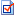Coordinate System Properties

Shows properties of the active coordinate system.

Coordinate system

Assigns the coordinate system to use to define the axis directions.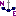Define Coordinate System by 3 Points

Defines a temporary coordinate system by three points. Point 1 defines the origin of the coordinate system. Point 2 defines the end of the local E-axis (X-axis). Point 3 defines the end of the local N-axis (Y-axis).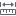Measure Distance Between 2 Points

Measures the linear distance between two key points. A key point is any point that you can highlight with the SmartSketch relationship indicators.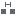Measure Minimum Distance

Measures the shortest distance between two objects.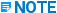The software does not use the SmartSketch list when measuring minimum distance. The SmartSketch list is used, however, when measuring distance.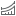Measure Distance Along Element

Measures from a reference point on an element to the last point clicked on the element, or along the entire element by pressing A on the keyboard. This mode is similar to the Point Along command.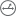Measure Radius and Diameter

Measures the diameter or radius of a hole or fillet.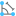Measure Angle Between 3 Points

Measures the angular value between three key points or two surfaces.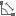Measure Angle Between Objects

Measures the angle between two objects in the model.

Plane

Measures the minimum distance between two objects as projected to a selected plane. The following options are available:

•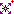No Plane - Measures the minimum distance between the two objects.

•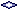Plan Plane - Calculates the apparent minimum distance between the two selected objects. The software calculates the minimum distance along the east and north coordinates and displays it on the ribbon bar. The minimum distances also displays in the graphic view using the annotation texts at the middle point of the solid yellow lines.

•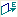Elevation Plane - East West - Calculates the minimum distance along the north and elevation coordinates and displays it on the ribbon bar. The distance also displays in the graphic view using the annotation texts at the middle point of the solid yellow lines.

•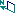Elevation Plane - North South - Calculates the minimum distance along the east and elevation coordinates and displays it on the ribbon bar. The distance also displays in the graphic view using the annotation texts at the middle point of the solid yellow lines.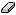Clear

Resets the Cumulative box to zero.

Cumulative

Sums repeated measurements until you clear this box or switch between angle and distance modes.

The remaining controls on the ribbon (defined below) depend on your selection of measuring distance, minimum distance, or angle.

Measuring Distance

Distance

Displays the distance value. You can specify the units by clicking Tools > Options and selecting the Units of Measure tab. For more information, see Change a displayed unit of measure.

D East (X)

Displays the distance along the E-axis (X-axis).

D North (Y)

Displays the distance along the N-axis (Y-axis).

D Elevation (Z)

Displays the distance along the EL-axis (Z-axis).

Measuring Minimum Distance

Minimum Distance

Displays the minimum distance value. You can specify the units by clicking Tools > Options and selecting the Units of Measure tab.

D E (X)

Displays the distance along the E-axis (X-axis).

D N (Y)

Displays the distance along the N-axis (Y-axis).

D EL (Z)

Displays the distance along the EL-axis (Z-axis).

Displays the radius of the hole or fillet.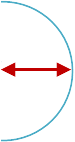Diameter

Displays the diameter of the object.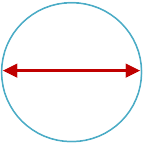Measuring Angles

Angle

Displays the angle measurement. You can specify the units by clicking Tools > Options and selecting the Units of Measure tab.

Apparent angle

Measures the angle of the lines projected onto the active view plane. If lines intersect (coplanar), then both the Angle and Apparent angle boxes display values. If lines do not intersect (are not coplanar), then only the Apparent angle box displays a value.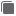Copy Measurement

Copies the measurement values from the ribbon.